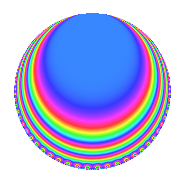# Properties

 Label 1800.1.u.aLevel 1800 Weight 1 Character orbit 1800.u Analytic conductor 0.898 Analytic rank 0 Dimension 4 Projective image $$D_{2}$$ CM/RM disc. -15, -120, 8 Inner twists 16

# Related objects

## Newspace parameters

 Level: $$N$$ = $$1800 = 2^{3} \cdot 3^{2} \cdot 5^{2}$$ Weight: $$k$$ = $$1$$ Character orbit: $$[\chi]$$ = 1800.u (of order $$4$$ and degree $$2$$)

## Newform invariants

 Self dual: No Analytic conductor: $$0.898317022739$$ Analytic rank: $$0$$ Dimension: $$4$$ Relative dimension: $$2$$ over $$\Q(i)$$ Coefficient field: $$\Q(\zeta_{8})$$ Coefficient ring: $$\Z[a_1, a_2]$$ Coefficient ring index: $$1$$ Projective image $$D_{2}$$ Projective field Galois closure of $$\Q(\sqrt{2}, \sqrt{-15})$$ Artin image size $$32$$ Artin image $OD_{16}:C_2$ Artin field Galois closure of $$\mathbb{Q}[x]/(x^{16} - \cdots)$$

## $q$-expansion

The $$q$$-expansion and trace form are shown below.

 $$f(q)$$ $$=$$ $$q$$ $$-\zeta_{8} q^{2}$$ $$+ \zeta_{8}^{2} q^{4}$$ $$-\zeta_{8}^{3} q^{8}$$ $$+O(q^{10})$$ $$q$$ $$-\zeta_{8} q^{2}$$ $$+ \zeta_{8}^{2} q^{4}$$ $$-\zeta_{8}^{3} q^{8}$$ $$- q^{16}$$ $$-2 \zeta_{8} q^{17}$$ $$-2 \zeta_{8}^{3} q^{23}$$ $$+ 2 q^{31}$$ $$+ \zeta_{8} q^{32}$$ $$+ 2 \zeta_{8}^{2} q^{34}$$ $$-2 q^{46}$$ $$+ 2 \zeta_{8} q^{47}$$ $$-\zeta_{8}^{2} q^{49}$$ $$-2 \zeta_{8} q^{62}$$ $$-\zeta_{8}^{2} q^{64}$$ $$-2 \zeta_{8}^{3} q^{68}$$ $$-2 \zeta_{8}^{2} q^{79}$$ $$+ 2 \zeta_{8} q^{92}$$ $$-2 \zeta_{8}^{2} q^{94}$$ $$+ \zeta_{8}^{3} q^{98}$$ $$+O(q^{100})$$ $$\operatorname{Tr}(f)(q)$$ $$=$$ $$4q$$ $$\mathstrut +\mathstrut O(q^{10})$$ $$4q$$ $$\mathstrut -\mathstrut 4q^{16}$$ $$\mathstrut +\mathstrut 8q^{31}$$ $$\mathstrut -\mathstrut 8q^{46}$$ $$\mathstrut +\mathstrut O(q^{100})$$

## Character Values

We give the values of $$\chi$$ on generators for $$\left(\mathbb{Z}/1800\mathbb{Z}\right)^\times$$.

 $$n$$ $$577$$ $$901$$ $$1001$$ $$1351$$ $$\chi(n)$$ $$\zeta_{8}^{2}$$ $$-1$$ $$1$$ $$1$$

## Embeddings

For each embedding $$\iota_m$$ of the coefficient field, the values $$\iota_m(a_n)$$ are shown below.

For more information on an embedded modular form you can click on its label.

Label $$\iota_m(\nu)$$ $$a_{2}$$ $$a_{3}$$ $$a_{4}$$ $$a_{5}$$ $$a_{6}$$ $$a_{7}$$ $$a_{8}$$ $$a_{9}$$ $$a_{10}$$
757.1
 0.707107 + 0.707107i −0.707107 − 0.707107i 0.707107 − 0.707107i −0.707107 + 0.707107i
−0.707107 0.707107i 0 1.00000i 0 0 0 0.707107 0.707107i 0 0
757.2 0.707107 + 0.707107i 0 1.00000i 0 0 0 −0.707107 + 0.707107i 0 0
1693.1 −0.707107 + 0.707107i 0 1.00000i 0 0 0 0.707107 + 0.707107i 0 0
1693.2 0.707107 0.707107i 0 1.00000i 0 0 0 −0.707107 0.707107i 0 0
 $$n$$: e.g. 2-40 or 990-1000 Significant digits: Format: Complex embeddings Normalized embeddings Satake parameters Satake angles

## Inner twists

Char. orbit Parity Mult. Self Twist Proved
1.a Even 1 trivial yes
8.b Even 1 RM by $$\Q(\sqrt{2})$$ yes
15.d Odd 1 CM by $$\Q(\sqrt{-15})$$ yes
120.i Odd 1 CM by $$\Q(\sqrt{-30})$$ yes
3.b Odd 1 yes
5.b Even 1 yes
5.c Odd 2 yes
15.e Even 2 yes
24.h Odd 1 yes
40.f Even 1 yes
40.i Odd 2 yes
120.w Even 2 yes

## Hecke kernels

This newform can be constructed as the kernel of the linear operator $$T_{7}$$ acting on $$S_{1}^{\mathrm{new}}(1800, [\chi])$$.